# Introduction To Numerical Analysis Using MATLAB with CD-ROM (Mathematics) (Computer Science) (Mathematics)

Numerical analysis is the branch of mathematics concerned with the theoretical foundations of numerical algorithms for the solution of problems arising in scientific applications. Designed for courses in numerical analysis and as a reference for practicing engineers and scientists, this book presents the theoretical concepts of numerical analysis and the practical justific Numerical analysis is the branch of mathematics concerned with the theoretical foundations of numerical algorithms for the solution of problems arising in scientific applications. Designed for courses in numerical analysis and as a reference for practicing engineers and scientists, this book presents the theoretical concepts of numerical analysis and the practical justification of these methods through computer examples with the latest version of MATLAB. The book addresses a variety of questions ranging from the approximation of functions and integrals to the approximate solution of algebraic, transcendental, differential and integral equations, with particular emphasis on the stability, accuracy, efficiency and reliability of numerical algorithms. The CD-ROM which accompanies the book includes source code, a numerical toolbox, executables, and simulations. FEATURES*Presents each numerical method by first providing examples and geometric motivation, then the steps to perform the computation, and finally the mathematical derivation of the process *Provides short programs in MATLAB that can be used for scientific applications with or without modifications *Shows the visual representation of mathematical concepts in 2D graphics and is compatible with the current MATLAB v.7.5. *Accompanied by a CD-ROM featuring source code, executables, figures, and simulations *Includes an introduction to MATLAB commands *Features an Instructor s Resource Disc for use as a textbook BRIEF TABLE OF CONTENTS 1. Number Systems and Errors. 2. Nonlinear Equations. 3. Systems of Linear Equations. 4. Approximating Functions. 5. Numerical Differentiation and Integration. 6. Ordinary Differential Equations. 7. The Eigenvalue problems. Appendix A. Mathematical Preliminaries. B. Introduction to MATLAB.

Compare

Numerical analysis is the branch of mathematics concerned with the theoretical foundations of numerical algorithms for the solution of problems arising in scientific applications. Designed for courses in numerical analysis and as a reference for practicing engineers and scientists, this book presents the theoretical concepts of numerical analysis and the practical justific Numerical analysis is the branch of mathematics concerned with the theoretical foundations of numerical algorithms for the solution of problems arising in scientific applications. Designed for courses in numerical analysis and as a reference for practicing engineers and scientists, this book presents the theoretical concepts of numerical analysis and the practical justification of these methods through computer examples with the latest version of MATLAB. The book addresses a variety of questions ranging from the approximation of functions and integrals to the approximate solution of algebraic, transcendental, differential and integral equations, with particular emphasis on the stability, accuracy, efficiency and reliability of numerical algorithms. The CD-ROM which accompanies the book includes source code, a numerical toolbox, executables, and simulations. FEATURES*Presents each numerical method by first providing examples and geometric motivation, then the steps to perform the computation, and finally the mathematical derivation of the process *Provides short programs in MATLAB that can be used for scientific applications with or without modifications *Shows the visual representation of mathematical concepts in 2D graphics and is compatible with the current MATLAB v.7.5. *Accompanied by a CD-ROM featuring source code, executables, figures, and simulations *Includes an introduction to MATLAB commands *Features an Instructor s Resource Disc for use as a textbook BRIEF TABLE OF CONTENTS 1. Number Systems and Errors. 2. Nonlinear Equations. 3. Systems of Linear Equations. 4. Approximating Functions. 5. Numerical Differentiation and Integration. 6. Ordinary Differential Equations. 7. The Eigenvalue problems. Appendix A. Mathematical Preliminaries. B. Introduction to MATLAB.

## 30 review for Introduction To Numerical Analysis Using MATLAB with CD-ROM (Mathematics) (Computer Science) (Mathematics)

1.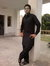5 out of 5

Bilal Ali

2.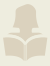4 out of 5

Vicky Arrozal

3.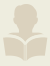5 out of 5

Ketan Sengar

4.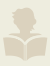5 out of 5

5.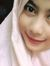5 out of 5

R Aprilia

6.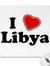4 out of 5

Mohammed Albishari

7.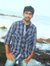5 out of 5

Bhanu Prakash

8.5 out of 5

Abuzar

9.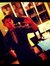5 out of 5

Árpád Bozi

10.4 out of 5

Pravesh

11.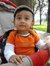5 out of 5

Shafaat Sheikh

12.4 out of 5

13.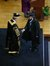5 out of 5

Syed Ghufran

14.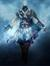4 out of 5

Ezio Auditore

15.4 out of 5

Prashanth Reddy

16.5 out of 5

Samsul Alam

17.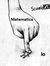4 out of 5

Sara Laera

18.4 out of 5

LingNing

19.4 out of 5

20.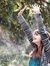5 out of 5

Sumarna

21.5 out of 5

Ahmed Elfeky

22.5 out of 5

Renuka Lakshmi

23.5 out of 5

Boubaker Krim

24.5 out of 5

Maysara

25.4 out of 5

26.4 out of 5

Davodm

27.5 out of 5

Nora

28.4 out of 5

Khoirun Nisa

29.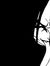5 out of 5

30.